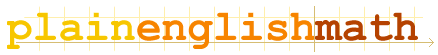You are here: MathematicsFundamentals → Functions

# Functions

If you've read the page on algebra in this section, then you already know about pronumerals; which are letters of the alphabet used to stand for variables (numbers which change depending on the situation concerned) in equations.

But what if a variable is itself dependent on another variable? Such variables are called functions (or occasionally, dependent variables), and the variables they depend on are called parameters (or independent variables, since the function depends on them).

## For example:

Imagine a scenario in which you have a sealed container sitting in an oven, and for some reason, you want to know the air pressure inside the container.
Let's make P stand for pressure and T be the temperature in degrees celsius. Suppose that the pressure is dependent solely on the temperature inside the container. This is written as P(T). In mathematical jargon, we'd say that "P is a function of T."
So P(T=0), or simply P(0), is equal to the pressure at zero degrees celsius, P(10) is the pressure at 10ºC and so on...
P(T) itself is simply equal to an expression, involving T, that evaluates to (i.e. is equal to) the pressure at temperature T.
So, for example, if P(T) = 5.66T + 300,
then P(0) = 5.66×0 + 300 = 300,
and P(10) = 5.66×10 + 300 = 56.6 + 300 = 356.6
Note that here, we're assuming that the sealed case has a rigid, non-flexible shape - in other words, the container's volume is constant. Otherwise, the container's pressure would be a function of both temperature and volume, and we'd write P(T, V).

## Composite functions

In the example above, what I didn't mention is that while P (pressure) is a function of T (temperature), the temperature inside the case is itself a function of how long the case has been inside the oven. Let's assume that this is the only parameter that affects the temperature inside the case (i.e. any others are carefully controlled and held constant). So, we can write T = T(t), where t is the elapsed time in seconds.

So, we have two functions; one, P(T), which gives pressure in terms of temperature, and another, T(t), that gives temperature in terms of time elapsed. We can actually combine the two together into one composite function to get pressure directly from the time elapsed.

We write: (P o T)(t)
P o T (read as "P composed with T") simply means "work out what T(t) equals, then work out P(T) using  and use the result as the parameter for the function. In other words, (P o T)(t) means P(T(t)).

## Powers of functions

Suppose you want to use a power of a function in an equation. For example, f(x) squared, or f(x) cubed. Never, ever, write this as f(x)2 or f(x)3, as this leads to ambiguity regarding exactly what is to be raised to the power - is it meant to be f(x2), f×(x)2 or (f(x))2? Mathematics should never contain such ambiguities! Instead, just write f2(x), which means "f squared, as a function of x," and is equivalent to writing (f(x))2.

## Conditions

Often, along with a function, there are associated conditions which restrict the possible parameters for that function. For example, the following function has the condition that its parameter, x, must be greater than or equal to 0:

f(x) = 4x2 + 7x + 6,    x ≥ 0

That means that f(x) is equal to 4x2 + 7x + 6 iff (iff means "if and only if") x ≥ 0.
What if x is less than zero? I haven't given a definition of what f(x) is equal to in this case, so it is said to be undefined. In other words, it doesn't make sense to look at what f(x) is equal to for x < 0, because this would break the x ≥ 0 relation and thus render the function nonsensical. If x < 0, f(x) simply isn't equal to anything. It's undefined.

But why can't x be less than zero for this function? In this case, I haven't given a reason. It's a hypothetical function with a hypothetical condition on its parameter. But there are many cases in the real world where similar conditions apply; for instance; if you ever have the opportunity to visit the south pole on foot, try walking further south then the south pole. You can't be a negative distance from the south pole! This is an example of a not-so-hypothetical condition on a parameter.

Sometimes; a function has two (or more) definitions - one for if a condition is met, and another which applies if the condition is not met (or a different condition is met). For example:

f(x) = {
 3x, x ≥ 0 x2, x < 0

Note that since f(x) can only be equal to a single number for a given value of x, it's important to write the conditions such that only one definition of f(x) applies for any one value of x. Otherwise, the two definitions would contradict each other.

Remember: functions act just like variables (and other numbers) in equations. The only difference is that they are themselves dependent on certain variables (parameters).